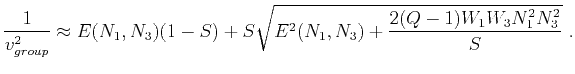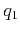On anelliptic approximations for qP velocities in TI and orthorhombic mediaNext: Proposed Approximations Up: Transversely isotropic media Previous: Muir and Dellinger Approximations

## Previous Approximations

To obtain more accurate approximations, Fomel (2004) suggested applying the shifted-hyperbola functional form, which introduces shift parameters(for phase velocity) and(for group velocity) into the approximations using the following functional form:(17)(18)

Parametersandcan be found by fitting the fourth derivativesandto the exact phase and group velocities, respectively. The results derived by Fomel (2004) for the vertical fitting (or) are(19)(20)

The introduction of parametersandleads to an increase in the number of parameters from three to four. To reduce this number back to three, Fomel (2004) suggested setting, or equivalently,, which results inand. Note that if we use equation 2 and set, this substitution will transform approximations 17 and 18 to the following form:(21)

and(22)

The phase-velocity approximation in equation 21 is equivalent to the acoustic approximation of Alkhalifah (1998,2000a) and the empirical approximation of Stopin (2001), which were derived in a different way. As discussed in the previous section, taking, or, equivalently,, is not necessarily the optimal choice, because the values of these two parameters may depend on the material properties of the media of interest. The actual empirical relationship betweenandcan be extracted from laboratory or in situ measurements of the stiffness tensor elements in various media (Figure 1). Furthermore, Equations 21 and 22 are derived by fitting the derivatives up to fourth-order at either the vertical or horizontal axis, whereas fitting at the other axis is only first-order. This low-order fitting may lead to a loss of accuracy at larger angles (or).On anelliptic approximations for qP velocities in TI and orthorhombic mediaNext: Proposed Approximations Up: Transversely isotropic media Previous: Muir and Dellinger Approximations

2017-04-14